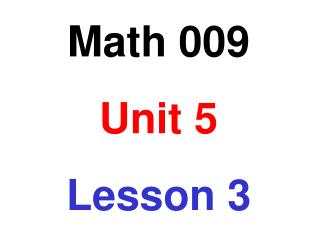DownloadDownload PresentationMath 009 Unit 5 Lesson 3

# Math 009 Unit 5 Lesson 3

Télécharger la présentation## Math 009 Unit 5 Lesson 3

- - - - - - - - - - - - - - - - - - - - - - - - - - - E N D - - - - - - - - - - - - - - - - - - - - - - - - - - -
##### Presentation Transcript

1. Math 009 Unit 5 Lesson 3

2. Obj: To write a ratio of two quantities in simplest form A ratio is a comparison of two quantities that have the same units. A ratio can be written in three different ways • As a fraction • As two numbers separated by a colon • As two numbers separated by the word to

3. Obj: To write a ratio of two quantities in simplest form Write the comparison 20 pounds to 24 pounds as a ratio in simplest form using a fraction, a colon and the word, to. 20 pounds 24 pounds 5 6 = = 5:6 = 5 to 6

4. Obj: To solve application problems involving ratios A company spends \$20,000 a month on television advertising and \$15,000 a month on radio advertising. What is the ratio in simplest form of the cost of radio advertising to the total amount spent for advertising? Total amount spent = \$35,000 radio advertising total 15,000 dollars 35,000 dollars 3 7 = =

5. Obj: To write rates A rate is a comparison of two quantities that have different units • the units are written as part of the rate Example: Write 15 pounds of fertilizer for 12 trees as a rate in simplest form. 5 pounds 4 trees 15 pounds 12 trees =

6. Obj: To write unit rates A unit rate is a rate in which the number in the denominator is 1 • to find a unit rate, divide the numerator of the rate by the denominator of the rate Example: Write 260 miles in 8 hours as a unit rate 3 2 .5 260 miles 8 hours = 32.5 miles/hour 8 260 .0 24 2 0 16 4 0 40

7. Obj: To solve application problems involving rates A jeweler purchased 5 ounces of gold for \$1625. Later, he sold the 5 ounces for \$1720. What was his profit per ounce? Total profit = 1720 – 1625 = \$95 profit ounce 95 dollars 5 ounces = = 19 dollars/ounce = \$19/oz

8. Obj: To solve application problems involving rates At one store John can buy 3 cans of corn for \$0.98. Another store has the same brand on sale 5 cans for \$1.67. Which is the better price? \$.98 3 cans \$ .327 /can = \$1.67 5 cans \$ .334 /can = 3 cans for \$0.98 is the better price